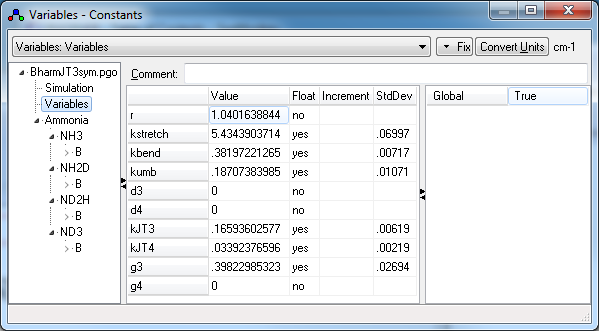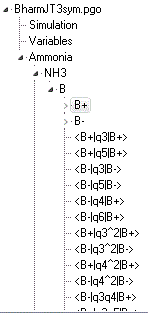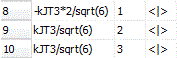Molecule Types Vibrational Structure Force Field Analysis Samples

# The Jahn-Teller effect in the B1E" state of NH3

This force field analysis includes the Jahn-Teller effect, and shows how isotope independent potential energy surface parameters can be used to calculate vibrational parameters and energy levels in NH3, NH2D, ND2H and ND3. The work is described in A.K. Saha, G. Sarma, C-H Yang, S. van de Meerakker, D.H. Parker and C.M. Western, Phys. Chem. Chem. Phys., (2015), doi:10.1039/C5CP01299F. There are 4 files:

• BSimpleHarm.pgo: A simple force field analysis for the B1E" state of NH3, ignoring Jahn-Teller effects. This is used to produce table 4 in the paper.
• BSimpleHarm.obs: The observation file used.
• BharmJT3sym.pgo: The multi-isotope model, with all 4 isotopologues and 2 electronic states for each.
• BharmJT3sym.obs: The observation file used to produce table 7 in the paper.

### Notes

• Neither .pgo file simulates a spectrum, as only one electronic state is set up in the file.
• The point group used throughout is C2v, even for NH3 and ND3 which are D3h. One of the consequences of this is that the modes are numbered 1..6, rather than the more normal 1..4. In the paper the numbering scheme 1, 2, 3x, 3y, 4x, 4y is used, corresponding to 1..6.
• The structure of the multi-isotopologue file is rather complex. At the upper levels it contains a variables object with the force constants, and a molecule object for each isotopologue, each of which has a single manifold object:• Each manifold object has two electronic state objects, B+ and B- (|+> and |-> in the paper), and multiple perturbation objects acting within and between the states. These are calculated from the force constants in the variables object controlled by settings in the internal coordinates object.• Each electronic state object has the normal z matrix, internal coordinate and symmetry coordinate objects typical of force field calculations. The  z matrix objects will only differ in the nuclear masses. The symmetry coordinates are all identical. The internal coordinate objects would all be identical were it not for the Jahn-Teller terms - see Other Potential Terms. Considering the linear Jahn-Teller terms in ν3 only, the B+ states have:This corresponds to equation (33) in the paper. Note the the first three terms contribute to perturbations acting within the B+ state, possibly including <B+|q1|B+>, <B+|q3|B+> and <B+|q3|B+>, depending on the isotopologue. The last two correspond to the second term in equation (33) and contribute to perturbations acting between the two electronic states, <B-|q4|B+>, <B-|q6|B+>. The B- states only have the terms acting within the electronic state, as the terms acting between states do not need to be duplicated:Note the sign changes compared to the B+ entry, reflecting the ± in equation (11).
• View, States will show the calculated energy levels - note that this is quite a time consuming calculation. The individual vmax entries have been adjusted to the minimum values converge the calculation.